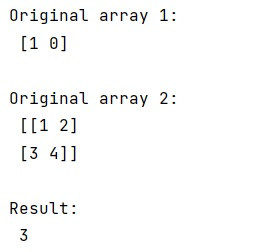# How to index a NumPy array with another NumPy array?

Learn, how can we index a NumPy array with another NumPy array in Python?
Submitted by Pranit Sharma, on March 02, 2023

NumPy is an abbreviated form of Numerical Python. It is used for different types of scientific operations in python. Numpy is a vast library in python which is used for almost every kind of scientific or mathematical operation. It is itself an array which is a collection of various methods and functions for processing the arrays.

## Indexing NumPy array with another NumPy array

Suppose that we are given two numpy arrays (say arr1 and arr2) and we need to index arr2 with arr1.

By indexing arr2 with another array, the arr2 must return the corresponding values. Also, arr1 must contain all the values less than or equal to the length of values of arr2. This can be simply done by converting the arr1 into a tuple and then indexing the arr2 with this tuple.

Let us understand with the help of an example,

## Python code to index a NumPy array with another NumPy array

```# Import numpy
import numpy as np

# Creating some numpy array
arr1 = np.array([1, 0])
arr2 = np.array([[1, 2],[3, 4]])

# Display original arrays
print("Original array 1:\n",arr1,"\n")
print("Original array 2:\n",arr2,"\n")

# Indexing arr2 with arr1
res = arr2[tuple(arr1.astype(int))]

# Display result
print("Result:\n",res,"\n")
```

Output:Languages: » C » C++ » C++ STL » Java » Data Structure » C#.Net » Android » Kotlin » SQL
Web Technologies: » PHP » Python » JavaScript » CSS » Ajax » Node.js » Web programming/HTML
Solved programs: » C » C++ » DS » Java » C#
Aptitude que. & ans.: » C » C++ » Java » DBMS
Interview que. & ans.: » C » Embedded C » Java » SEO » HR
CS Subjects: » CS Basics » O.S. » Networks » DBMS » Embedded Systems » Cloud Computing
» Machine learning » CS Organizations » Linux » DOS
More: » Articles » Puzzles » News/Updates